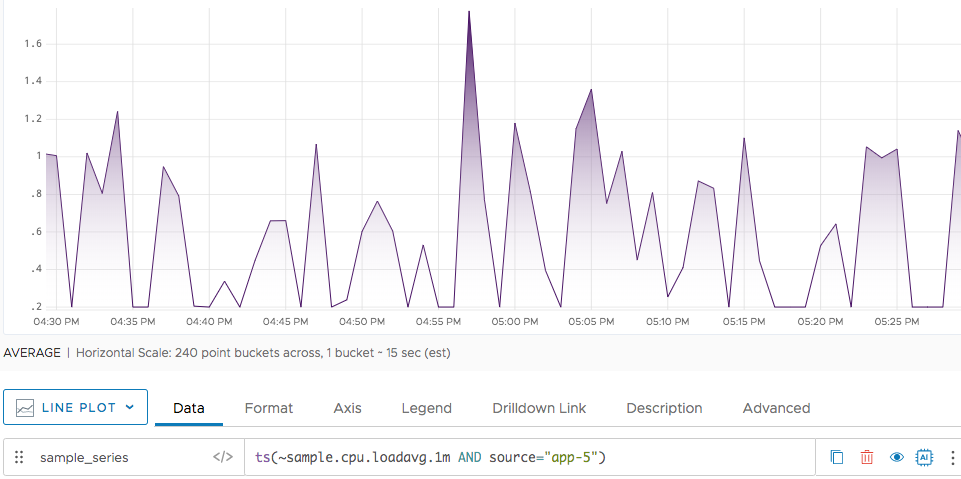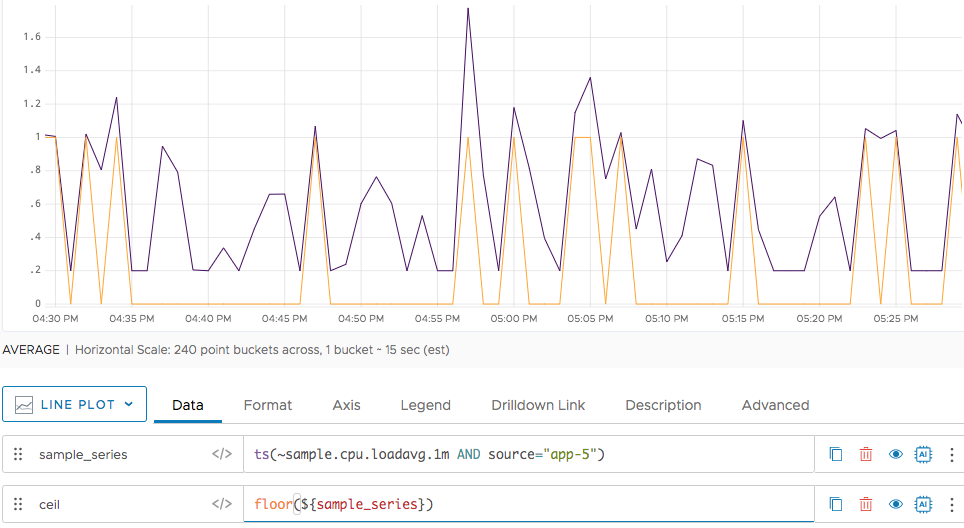Reference to the floor() function

## Summary

``````floor(<tsExpression>)
``````

Returns the floor for the time series described by the expression, by rounding any data values with decimals down to the nearest integer.

## Parameters

ParameterDescription
tsExpression Expression describing the time series to return floor values for.

## Description

The `floor()` function returns the floor for each data value in the time series described by the expression.

The floor of a data value is the greatest integer that is less than or equal to that value:

Sample query with floor()Sample resultInput data valueReturned value (floor)
`floor(1.75)` 1 Positive, with fractional partInteger part of the input value
`floor(-1.75)` -2 Negative, with fractional partNext lower integer
`floor(1)` 1 Positive integerSame as the input value
`floor(-1)` -1 Negative integerSame as the input value

`floor()` returns a separate series of results for each time series described by the expression.

## Examples

Here’s a query that returns a time series that reports positive fractional values between .2 and 1.2. We’ve changed the color to a dark purple.Now we apply `floor()` to our original query. Notice that the values below 1 are mapped to 0, and the values from 1 to 1.2 are mapped to 1. The color for the `floor()` time series is orange.`ceil()`
`round()`